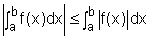Ch 6. Integrals Multimedia Engineering Math IndefiniteIntegral Area DefiniteIntegral FundamentalTheorem SubstitutionRule
 Chapter 1. Limits 2. Derivatives I 3. Derivatives II 4. Mean Value 5. Curve Sketching 6. Integrals 7. Inverse Functions 8. Integration Tech. 9. Integrate App. 10. Parametric Eqs. 11. Polar Coord. 12. Series Appendix Basic Math Units Search eBooks Dynamics Fluids Math Mechanics Statics Thermodynamics Author(s): Hengzhong Wen Chean Chin Ngo Meirong Huang Kurt Gramoll ©Kurt GramollMATHEMATICS - THEORY This section introduces the definition of the integral and its properties. Definite Integral In Area section, the limit was used to approximate the area between a curve and x-axis. This type of limit often occurs in engineering research, such as finding the fluid pressure over an area or the work used in a process. A special name and notation is given to this kind of limit - definite integral. It states: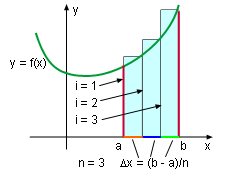Definite Integral If a function f is defined on a closed interval between a and b, and it is equally divided into n parts. So the length of each part is Δx = (b-a)/n, then the definite integral of function f from a to b is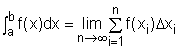(1) In this equation, ∫ is the integration sign; a is the lower limit; b is the upper limit; f(x) is the integrand. The procedure for calculating an integral is called integration and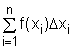is called the Riemann sum. The definite integral is sometimes called the Riemann integral. The definite integral of the function f(x),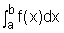, is a number. When the function is positive, an integral can be interpreted as an area.= the area under the graph of function from a to b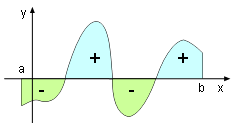Integral and area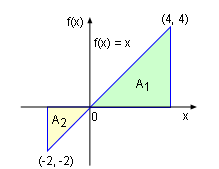Integral and area In general, a definite integral can be interpreted as the difference of areas: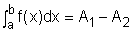in which A1 is the sum area of the region above the x-axis and A2 is the sum area of the region below the x-axis as shown on the left. For example, the integral function f(x) = x between -2 and 4 can be calculated as the difference of the the two triangles.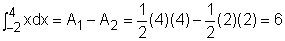Although this problem can be calculated, some of the integral can't be calculated. IntegrableIntegrable Discontinuous Function It is clear that, if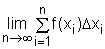exist, then the function f(x) is integrable. The prerequisite forexisting is the function f(x) must be continuous or monotonic. Therefore, if a function is either continuous or monotonic on a close interval between a and b, then the function is integrable on this interval. If the function is discontinuous, its integral might exist, such as the step function shown on the left. Any function that is integrable between a and b can be expressed as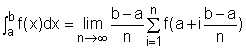Midpoint Rule In order to calculate the limit, the right endpoints are generally used. If the midpoint is used, the limit will be an approximate value and the integral will be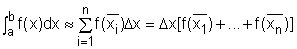where Δx = (b - a)/n and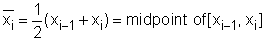To assist in evaluating integrals, some basic properties of integrals are list below. Properties of the Integral If the integral of a function exist in [a, b], then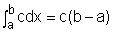where c is any constant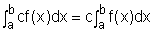where c is any constant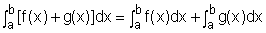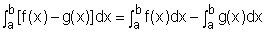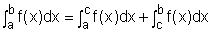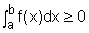if f(x) ≥0.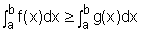if f(x) ≥g(x).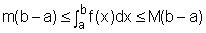if m ≤ f(x) ≤ M .